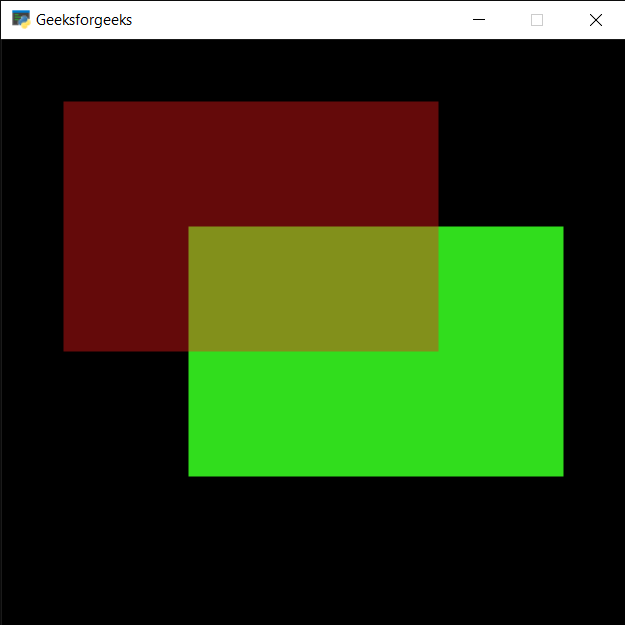# PYGLET – Drawing Rectangle

• Last Updated : 09 Jul, 2021

In this article we will see how we can draw rectangle on window in PYGLET module in python. Pyglet is easy to use but powerful library for developing visually rich GUI applications like games, multimedia etc. A window is a “heavyweight” object occupying operating system resources. Windows may appear as floating regions or can be set to fill an entire screen (fullscreen). A rectangle is a four-sided shape where every angle is a right angle (90°). Also opposite sides are parallel and of equal length. Rectangle is drawn with the help of shapes module in pyglet.
We can create a window with the help of command given below

```# creating a window
window = pyglet.window.Window(width, height, title)```

In order to create window we use Rectangle method with pyglet.shapes
Syntax : shapes.Rectangle(x, y, width, height, color=(255, 255, 255), batch=None)
Argument : It takes position i.e pair of integer, width and height of rectangle, color of rectangle and last is batch object
Return : It returns Line object

Below is the implementation

## Python3

 `# importing pyglet module``import` `pyglet` `# importing shapes from the pyglet``from` `pyglet ``import` `shapes` `# width of window``width ``=` `500``  ` `# height of window``height ``=` `500``  ` `# caption i.e title of the window``title ``=` `"Geeksforgeeks"``  ` `# creating a window``window ``=` `pyglet.window.Window(width, height, title)` `# creating a batch object``batch ``=` `pyglet.graphics.Batch()`  `# properties of rectangle``# co-ordinates of rectangle``co_x ``=` `150``co_y ``=` `150` `# width of rectangle``width ``=` `300` `# height of rectangle``height ``=` `200` `# color = green``color ``=` `(``50``, ``225``, ``30``)` `# creating a rectangle``rec1 ``=` `shapes.Rectangle(co_x, co_y, width, height, color ``=` `color, batch ``=` `batch)` `# changing opacity of the rect1``# opacity is visibility (0 = invisible, 255 means visible)``rec1.opacity ``=` `250`  `# creating another rectangle with properties``# x, y co ordinate : 50, 250``# width, height of rectangle : 300, 200``# color = red``color ``=` `(``255``, ``25``, ``25``)` `# creating rectangle``rec2 ``=` `shapes.Rectangle(``50``, ``250``, ``300``, ``200``, color ``=` `color, batch ``=` `batch)` `# changing opacity of the rec2``# opacity is visibility (0 = invisible, 255 means visible)``rec2.opacity ``=` `100`  `# window draw event to draw rectangles``@window``.event``def` `on_draw():``    ` `    ``# clear the window``    ``window.clear()``    ` `    ``# draw the batch``    ``batch.draw()` `# run the pyglet application``pyglet.app.run()`My Personal Notes arrow_drop_up